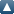# Items where Author is "Kolokoltsov, VN"Up a level
 Export as ASCII CitationBibTeXDublin CoreEP3 XMLEndNoteHTML CitationJSONMETSMultiline CSVObject IDsOpenURL ContextObjectRDF+N-TriplesRDF+N3RDF+XMLRefWorksReferReference Manager
Group by: Item Type | No Grouping
Number of items: 18.

## Journal article

KOLOKOLTSOV, V.N., SCHILLING, R.L. and TYUKOV, A.E., 2004. Estimates for multiple stochastic integrals and stochastic Hamilton-Jacobi equations. Revista Matematica Iberoamericana, 20 (2), pp. 333-380. ISSN 0213-2230

KOLOKOLTSOV, V.N., 2004. Hydrodynamic limit of coagulation-fragmentation type models of k-nary interacting particles. Journal of Statistical Physics, 115, pp. 1621-1653. ISSN 0022-4715

KOLOKOLTSOV, V.N., 2004. Measure-valued limits of interacting particle systems with k-nary interaction II. Finite-dimensional limits. Stochastics and Stochastics Reports, 76 (1), pp. 45-58.

KOLOKOLTSOV, V.N., 2004. On Markov processes with decomposable pseudo-differential generators. Stochastics and Stochastics Reports, 76 (1), pp. 1-44.

KOLOKOLTSOV, V.N., 2003. Measure-valued limits of interacting particle systems with k-nary interactions - I. One-dimensional limits. Probability Theory and Related Fields, 126 (3), pp. 364-394. ISSN 0178-8051

BELAVKIN, V.P. and KOLOKOLTSOV, V.N., 2003. On a general kinetic equation for many-particle systems with interaction, fragmentation and coagulation. Proceedings of the Royal Society of London Series A-Mathematical Physical and Engineering Sciences, 459 (2031), pp. 727-748. ISSN 1364-5021

KOLOKOLTSOV, V.N., 2003. On extensions of mollified Boltzmann and Smoluchowski equations to particle systems with a k-ary interaction. Russian Journal of Mathematical Physics, 10 (3), pp. 268-295. ISSN 1061-9208

KOLOKOLTSOV, V.N., 2003. On the singular Schroedinger equations with magnetic fields. Matematicheskii Sbornik, 194 (6), pp. 105-126. ISSN 0368-8666

KOLOKOLTSOV, V.N., 2003. Schrodinger operators with singular potentials and magnetic fields. Sbornik Mathematics, 194, pp. 897-917. ISSN 1064-5616

KOLOKOLTSOV, V.N. and TYUKOV, A.E., 2003. Small time and semiclassical asymptotics for stochastic heat equations driven by Levy noise. Stochastics and Stochastics Reports, 75, pp. 1-38.

BELAVKIN, V.P. and KOLOKOLTSOV, V.N., 2002. Stochastic evolution as a quasiclassical limit of a boundary value problem for Schrodinger equations. Infinite Dimensional Analysis, Quantum Probability and Related Topics, 5 (1), pp. 61-91. ISSN 0219-0257

KOLOKOLTSOV, V.N., SCHILLING, R.L. and TYUKOV, A.E., 2002. Transience and non-explosion of certain stochasitic Newtonian systems. Electronic Journal of Probability, 7.

KOLOKOLTSOV, V.N., 2002. A new path integral representation for the solutions of the Schrodinger, heat and stochastic Schrodinger equations. Mathematical Proceedings of the Cambridge Philosophical Society, 132, pp. 353-375. ISSN 0305-0041

KOLOKOLTSOV, V.N., KOROLEV, V. and UCHAIKIN, V., 2001. Fractional stable distributions. Journal of Mathematical Sciences, 105 (6), pp. 2569-2576. ISSN 1072-3374

KOLOKOLTSOV, V.N., 2001. Small diffusion and fast dying out asymptotics for superprocesses as non-Hamiltonian quasiclassics for evolution equations. Electronic Journal of Probability, 6. ISSN 1083-6489

KOLOKOLTSOV, V.N., 2001. The rate of escape of stable Ornstein-Uhlenbeck processes and the scattering theory for thier perturbations. Markov Processes and Related Fields, 7 (4), pp. 603-626.

## Conference contribution

KOLOKOLTSOV, V.N., 2001. Mathematics of the Feynmann path integral. In: Proceedings of the International Mathematical Conference FILOMAT 2001, Nis, Serbia.

BELAVKIN, V.P. and KOLOKOLTSOV, V.N., 2001. Stochastic evolutions as boundary value problems. In: RYMS Kokyuroku 1227: Infinite Dimensional Analysis and quantum Probability Theory, Kyoto, Japan.

This list was generated on Fri Sep 22 00:51:09 2023 UTC.